# 泰勒级数

 泰勒级数展开 总和符号记法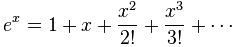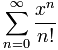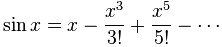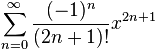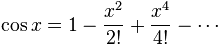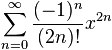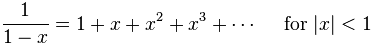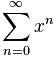（还有很多）

## 近似值

 1 − x2/2!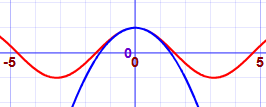1 − x2/2! + x4/4!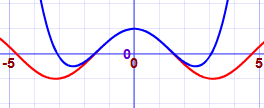1 − x2/2! + x4/4! − x6/6!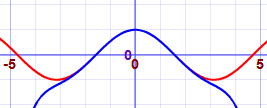1 − x2/2! + x4/4! − x6/6! + x8/8!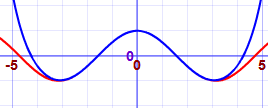（你可以在 复数的欧拉公式 看到更多泰勒级数的用法。）

## 背后的理念是什么？

f(x) = c0 + c1(x-a) + c2(x-a)2 + c3(x-a)3 + ……

### 简短回顾：导数告诉我们函数在任何一点的坡度。

• 常数 的导数是 0
• x 的导数是 1
• xn 的导数是 nxn-1 （例子：x3 的导数是 3x2)

f(a) = c0 的项都等于零

f(x) = c1 + 2c2(x-a) + 3c3(x-a)2 + ……

f(a) = c1

f(x) = 2c2 + 3×2×c3(x-a) + ……

f(a) = 2c2

• 高一个阶的导数……
• ……除以在这项以下所有的指数的积（我们可以用 阶乘记号 来写，例如 3! = 3×2×1）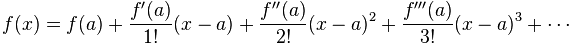### 例子：cos(x) 的泰勒级数

• cos(x) 的导数是 -sin(x)
• sin(x) 的导数是 cos(x)

• c0 = f(0) = cos(0) = 1
• c1 = f'(0)/1! = -sin(0) = 0
• c2 = f''(0)/2! = -cos(0)/2! = -1/2!
• c3 = f'''(0)/3! = sin(0)/3! = 0
• c4 = f''''(0)/4! = cos(0)/4! = 1/4!
• 等等……

cos(x) = 1 − x2/2! + x4/4! −……